# If a balloon has a volume of 1.329 L at a pressure of 2.265 atmospheres, what...

If a balloon has a volume of 1.329 L at a pressure of 2.265 atmospheres, what will be the volume of the balloon if the pressure is increased to 4.993 atmospheres?

1.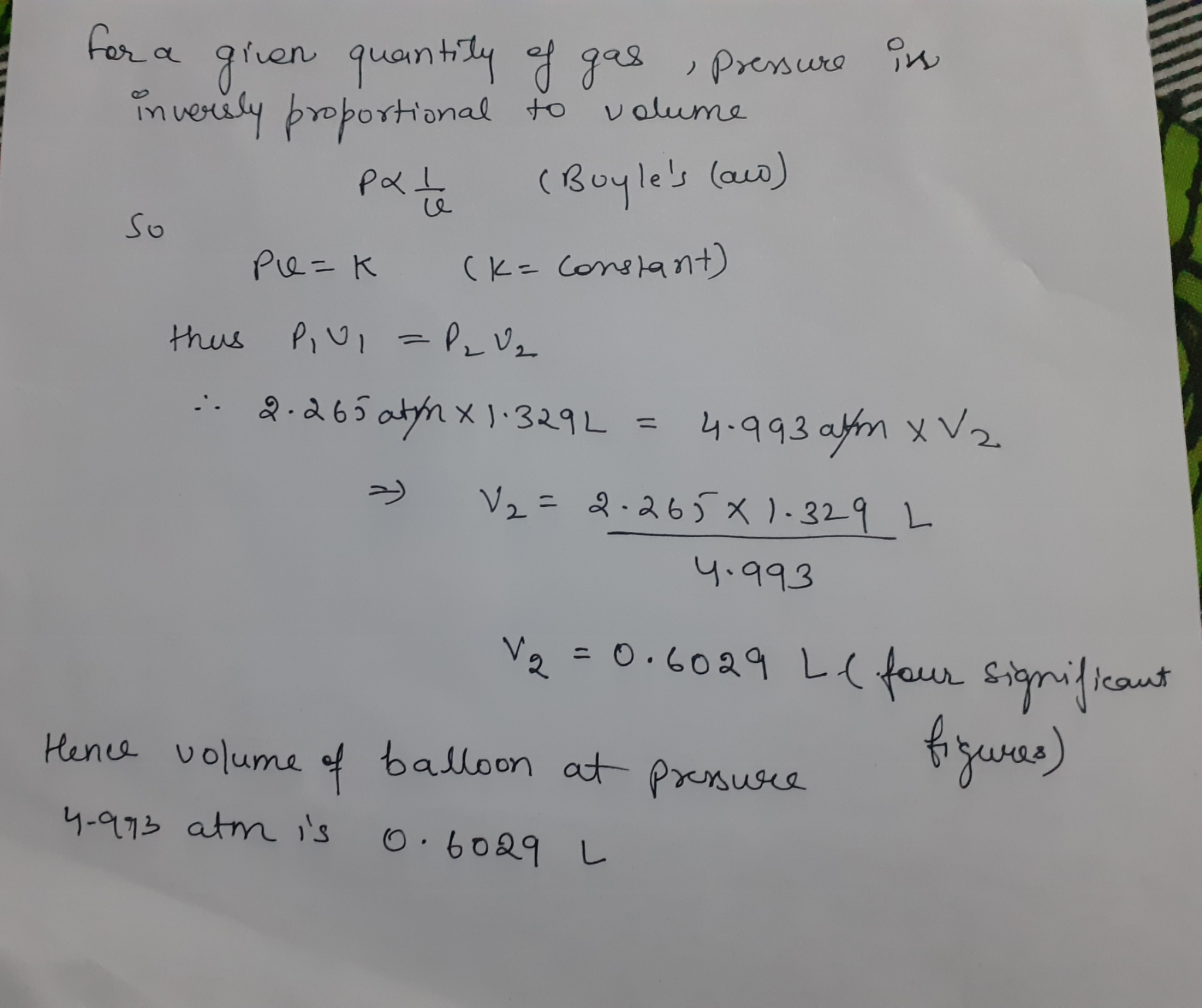SOLUTION :

As per Boyle’s Law,

P V = constant

So,

P1 V1 = P2 V2

=> V2 = (P1/P2) * V1

=> V2 = (2.265 / 4.993) * 1.329

=> V2 = 0.6029 L

So, volume of balloon at increased pressure of 4.993 atm  will be 0.6029 L (ANSWER).

#### Earn Coin

Coins can be redeemed for fabulous gifts.

Similar Homework Help Questions
• ### A helium-containing balloon has a volume of 10.6 L and a pressure of 1.22 atm at...

A helium-containing balloon has a volume of 10.6 L and a pressure of 1.22 atm at a temperature of 27.9C. What will the pressure inside the balloon be if the temperature is lowered to -11.3C? Assume that the gas behaves ideally. Express your answer in units of atmospheres (atm) using at least three significant figures.

• ### A 1.8 L volume of neon gas (monatomic) is at a pressure of 4.0 atmospheres and...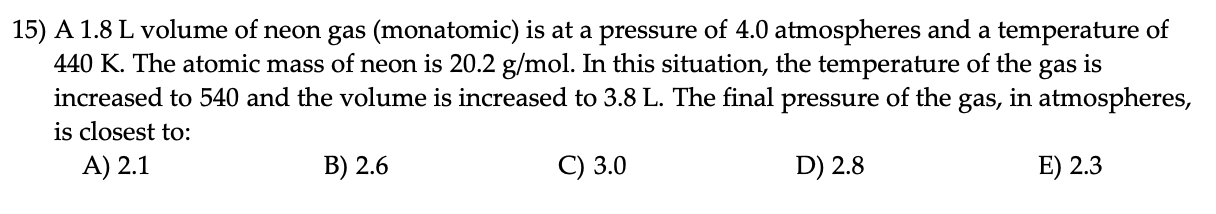A 1.8 L volume of neon gas (monatomic) is at a pressure of 4.0 atmospheres and a temperature of 440 K. The atomic mass of neon is 20.2 g/mol. In this situation, the temperature of the gas is increased to 540 and the volume is increased to 3.8 L. The final pressure of the gas, in atmospheres, is closest to: A) 2.1 B) 2.6 C) 3.0 D) 2.8 E) 2.3 15) A 1.8 L volume of neon gas (monatomic) is...

• ### A gas with a pressure of 36 atm and a volume of 22.4 L is held...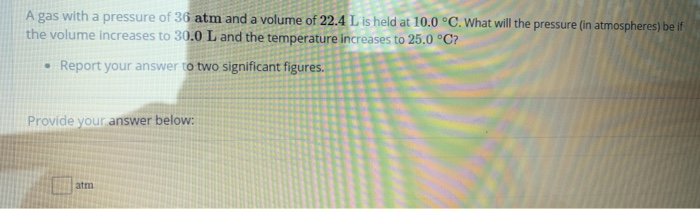A gas with a pressure of 36 atm and a volume of 22.4 L is held at 10.0 °C. What will the pressure (in atmospheres) be if the volume increases to 30.0 L and the temperature increases to 25.0 °C? • Report your answer to two significant figures Provide your answer below:

• ### A weather balloon is filled with helium to a volume of 350 L at 12 ∘C...

A weather balloon is filled with helium to a volume of 350 L at 12 ∘C and 747 mmHg . The balloon ascends to an altitude where the pressure is 499 mmHg and the temperature is -36 ∘C What is the volume of the balloon at this altitude? Express your answer using three significant figures.

• ### Problem 7. The pressure inside a 1.0L balloon at 25 in atmospheres, when the balloon is...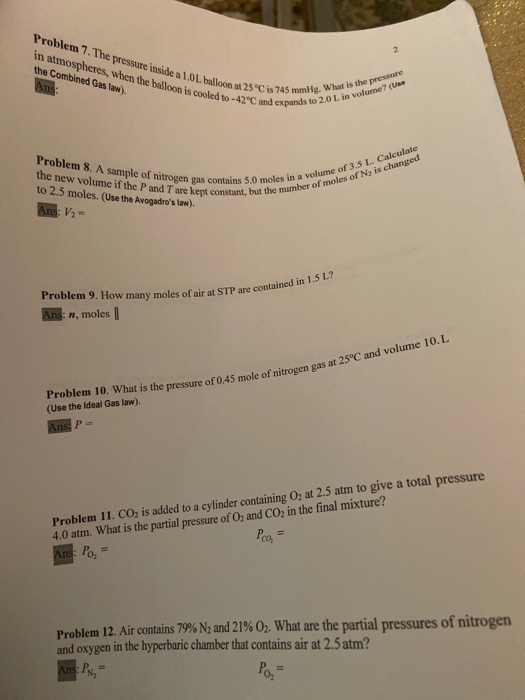Problem 7. The pressure inside a 1.0L balloon at 25 in atmospheres, when the balloon is cooled to -42°C the Combined Gas law). Ans: is the pressure is 745 mmHe What is the pro expands to 2.0 L in volume Problem 8. A sample of nitrogen gas contains the new volume if the P and Tare kept constant, but to 2.5 moles. (Use the Avogadro's law). Ans: V2 ° 2.5 moolume if pleoni O moles in a volume of 3.5...

• ### 007 10.0 points A helium balloon has a volume of 21.8 L when the pressure is...007 10.0 points A helium balloon has a volume of 21.8 L when the pressure is 0.578 atm and the tem- perature is 19°C. The balloon is cooled at a constant pressure until the temperature is -11°C. What is the volume of the balloon at this stage? Answer in units of L.

• ### A weather balloon is filled with helium to a volume of 270 L at 29 ∘C...

A weather balloon is filled with helium to a volume of 270 L at 29 ∘C and 765 mmHg . The balloon ascends to an altitude where the pressure is 466 mmHg and the temperature is -42 ∘C. You may want to reference (Pages 233 - 234) Section 8.8 while completing this problem. Part A What is the volume of the balloon at this altitude? Express your answer using three significant figures.

• ### The air in a 6.00 L tank has a pressure of 2.00 atm . What is...

The air in a 6.00 L tank has a pressure of 2.00 atm . What is the final pressure, in atmospheres, when the air is placed in tanks that have the following volumes, if there is no change in temperature and amount of gas? Part A 1.20 L Express your answer using three significant figures. P1 P 1 =      atm   SubmitRequest Answer Part B 2300. mL Express your answer using four significant figures. P2 P 2 =   atm   SubmitRequest...

• ### The pressure with a 9.69 L balloon is 8.92 atm. If the volume of the balloon increases to 9.9 L, what will be the f...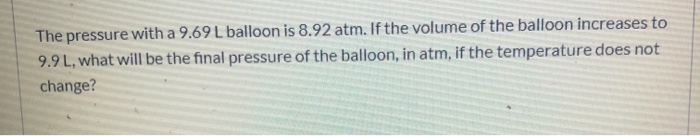The pressure with a 9.69 L balloon is 8.92 atm. If the volume of the balloon increases to 9.9 L, what will be the final pressure of the balloon, in atm, if the temperature does not change?

• ### Learning Goal: To apply the ideal gas law to problems involving temperature, pressure, volume, and moles...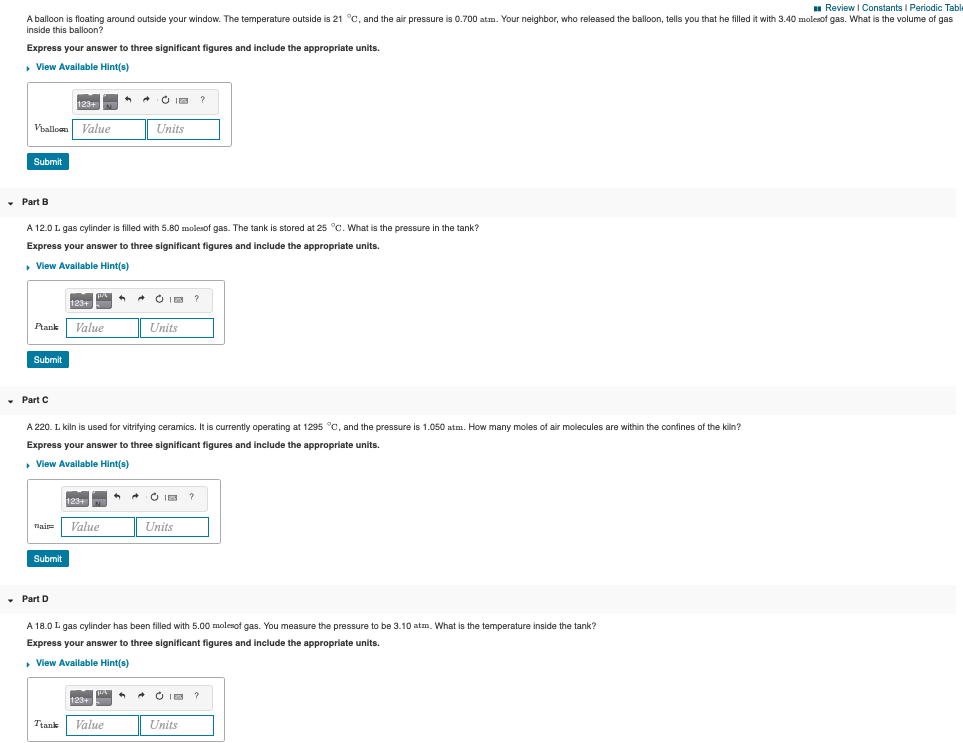Learning Goal: To apply the ideal gas law to problems involving temperature, pressure, volume, and moles of a gas. The four properties of gases (pressure P, volume V, temperature T, and moles of gas n) are related by a single expression known as the ideal gas law: PV=nRT The variable R is known as the universal gas constant and has the value R=0.0821 L⋅atm/(mole⋅K). The units of R dictate the units for all other quantities, so when using this value...